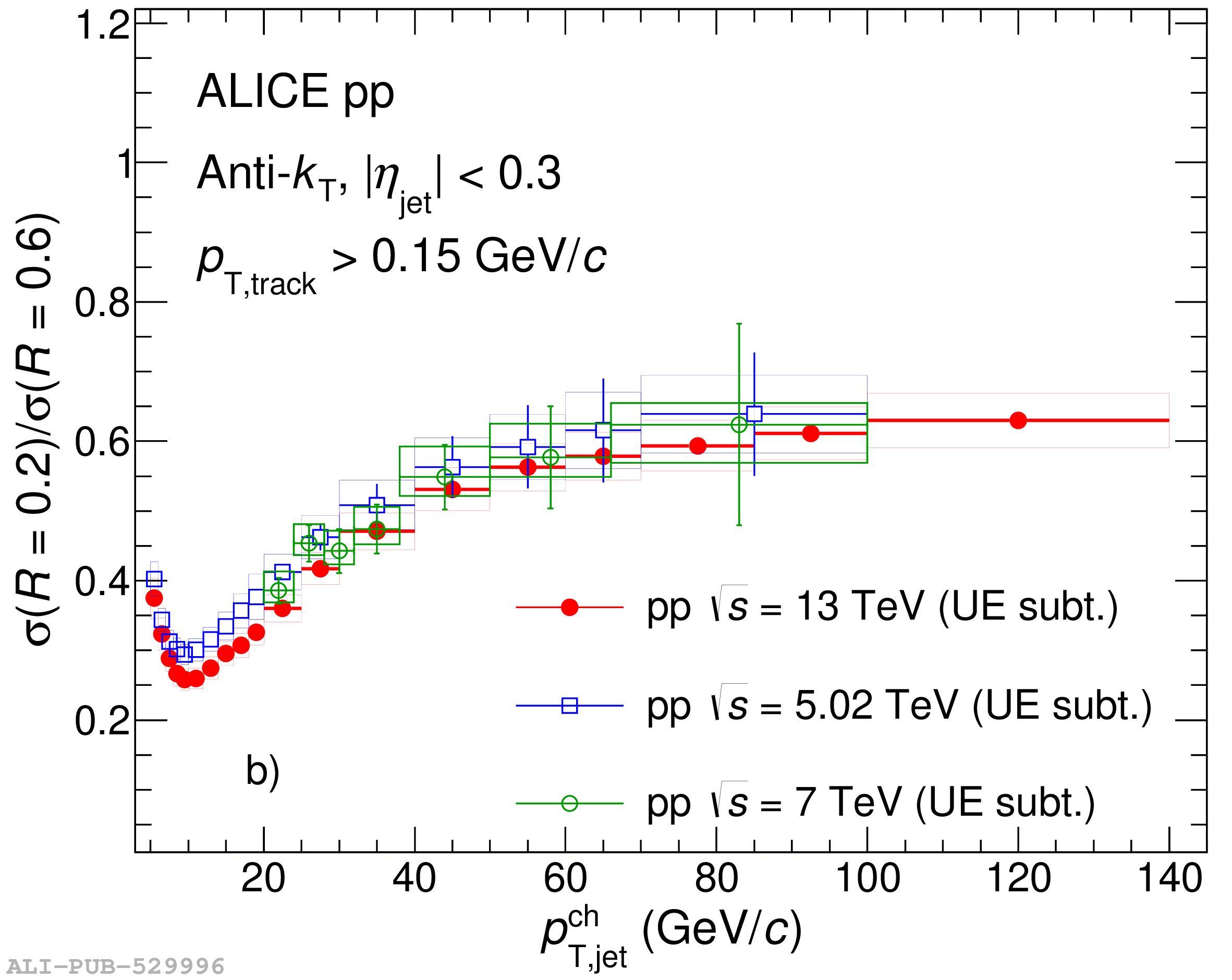# Figure 6

 Comparison of charged-particle jet cross section ratio with UE subtraction in pp collisions at $\sqrt{s} = 5.02$ , $7$ , and $13$ $\mathrm{TeV}$ and in p--Pb collisions at $\sqrt{s_\mathrm{NN}} = 5.02 \ \mathrm{TeV}$ . Results are a) $\sigma(R=0.2)/\sigma(R=0.4)$ , and b) $\sigma(R=0.2)/\sigma(R=0.6)$.